• Call Now

1800-102-2727•

Chemical Composition - Definition of Chemical Composition, Law of Constant Composition, Practice Problems and FAQs

Have you ever wondered why we use a particular hand sanitiser, soap or hand wash?What is the factor which helps you in taking decisions while buying such a product? Well, the answer is “Chemical Composition”. It has been always advised to look for chemical composition before buying any product. Every compound has a specific ratio of its constituent.

Let’s understand the chemical composition of compounds in more detail!

Table of Content

• What is a Chemical Composition of a Compound?
• Determination of Chemical Composition
• Law of Constant Proportions
• Practice Problems
• Frequently Asked Questions - FAQs

## What is a Chemical Composition of a Compound?

Chemical composition is referred to the proportions of atoms in a molecule of a chemical substance.

So, basically, it is the percentage proportion of any particular element in a compound.

The chemical composition determines the physical and chemical properties of the substance. It can be concluded that composition defines the way atoms are put together. Density, colour, strength and other properties are associated with the substance. Any substance which has a specific structure and chemical composition is known as a chemical. Chemicals are known to exist in a range of states i.e., they can be either in solids, liquids or gases. Their states can be changed and are influenced by certain factors like pressure, temperature etc.

## Determination of Chemical Composition

Calculating the chemical composition of a substance is calculated in terms of percentage. The percentage defines the contribution of each element in the substance by its mass. The steps to calculate the chemical composition of elements present in the compound are given below:

• The initial step is to determine the atomic mass of all the elements present in the compound.
• Next step is to determine the molecular mass of the compound as a whole.
• Divide the atomic mass of the element with the molecular mass of the compound.
• The number resulting from division results in a value less than 1. Multiply this number with 100 in order to determine the percentage of composition of the compound.

For example, the molecular formula of pentane is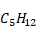. The percentage composition of pentane is calculated using the following procedure:

Mass of hydrogen per molecule of pentane: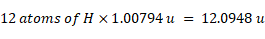Mass of carbon per molecule of pentane: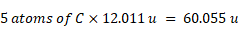Molecular mass of pentane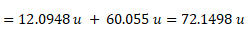Mass % of hydrogen in pentane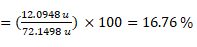Mass % of carbon in pentane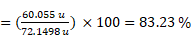Law of Constant Proportions

The law of constant proportions was given by French chemist, Joseph Louis Proust in 1779. This law is also known as the Law of Constant Proportions.

According to this law, a compound always contains exactly the same proportion of elements by weight irrespective of their source or method of preparation.

For example, in the molecule of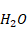, Hydrogen, and Oxygen combine in a 1: 8 weight ratio, this ratio remains constant whether it is mineral water, seawater or produced by any other chemical reaction (For example: a by-product of neutralization reaction).

Take another example for reference:

The combustion of 7.25 g of a certain metal in the presence of oxygen produced 10.15 g of its oxide. When heated in steam, 9 g of the same metal produced 12.6 g of its oxide. Demonstrate how these findings support the law of constant proportions.

In case-1:

Weight of metal = 7.25 g

Weight of oxygen = (10.15-7.25) = 2.9 g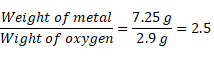In case-2:

Weight of metal = 9 g

Weight of oxygen = (12.6 - 9) = 3.6 g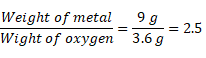Thus, in both the cases, the proportions of the weight of metal and oxygen is fixed as the ratio is same in both the cases. Hence, both these findings support the law of definite proportions.

## Practice Problems

Q1. Which of the following scientists gave the idea of the law of constant composition?

1. Joseph Proust
3. Neil Bohr
4. John Dalton

Solution: The law of constant composition was given by chemist Joseph Proust. The conclusion drawn by Proust is that all samples of a compound have the same composition, or the same proportion, by mass, of the compound's constituent elements. For instance, water is primarily a chemical compound composed of the two elements hydrogen and oxygen in a specific proportion.

Q2. IUPAC stands for:

1. Indian Union of Pure and Applied Chemistry
2. International Union of Pure and Applied Chemistry
3. Indonesian Union of Pure and Applied Chemistry
4. International Union of Practical and Applied Chemistry

Solution: IUPAC stands for International Union of Pure and Applied Chemistry (IUPAC).

Q3. What is the mass percentage composition of methane?

1.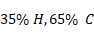2.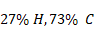3.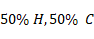4.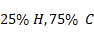Solution:

Methane has a molecular formula of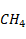Molecular mass of methane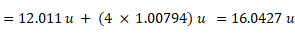Mass % of hydrogen in methane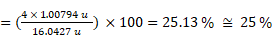Mass % of carbon in methane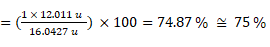Hence, percentage composition of methane is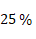hydrogen and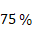carbon.

Q4. What is the mass percentage composition of ammonia?

1.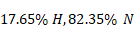2.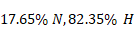3.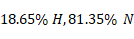4.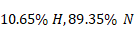Solution:

Ammonia has a molecular formula of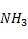Molecular mass of ammonia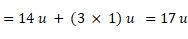Mass % of hydrogen in ammonia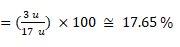Mass % of carbon in ammonia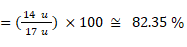Hence, percentage composition of ammonia is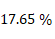hydrogen and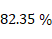nitrogen.

## Frequently Asked Questions - FAQs

Q.1 What is the purpose of the law of definite proportions?

Answer: The law of definite proportions ensures that chemical compounds are always created using the same proportions, regardless of the amount of the compound which is being made.

Q.2 What type of matter does not follow the law of constant proportions?

Answer: No, the Law of Definite Proportions applies only to compounds. When two or more elements are chemically bonded together, a compound is created.

Q.3 Do chemical reactions proceed under the law of definite proportions?

Answer: The law of definite proportions states that a chemical compound always contains the same elements in exactly the same proportions by mass, was quickly discovered after it was discovered that mass was always conserved in chemical reactions.

Q.4 Why do mixtures not obey the law of constant composition?

Answer: A mixture is not chemically united, only physically. You can put as much of one thing in with the other because there is no limit, they are only mixing physically. Compounds, or elements that are chemically combined, are subject to the law of definite proportions.

Related TopicsTalk to our expert
Resend OTP Timer =
By submitting up, I agree to receive all the Whatsapp communication on my registered number and Aakash terms and conditions and privacy policy